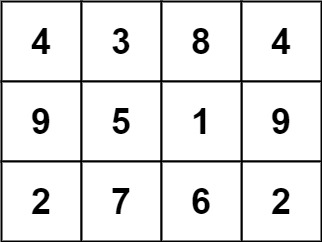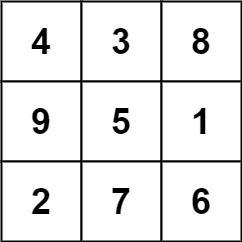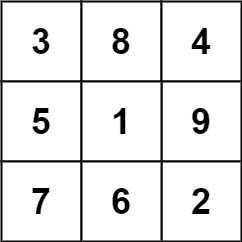840. Magic Squares In Grid

A `3 x 3` magic square is a `3 x 3` grid filled with distinct numbers from `1` to `9` such that each row, column, and both diagonals all have the same sum.

Given a `row x col` `grid` of integers, how many `3 x 3` "magic square" subgrids are there?  (Each subgrid is contiguous).

Example 1:```Input: grid = [[4,3,8,4],[9,5,1,9],[2,7,6,2]]
Output: 1
Explanation:
The following subgrid is a 3 x 3 magic square:while this one is not:In total, there is only one magic square inside the given grid.
```

Example 2:

```Input: grid = []
Output: 0
```

Example 3:

```Input: grid = [[4,4],[3,3]]
Output: 0
```

Example 4:

```Input: grid = [[4,7,8],[9,5,1],[2,3,6]]
Output: 0
```

Constraints:

• `row == grid.length`
• `col == grid[i].length`
• `1 <= row, col <= 10`
• `0 <= grid[i][j] <= 15`

840. Magic Squares In Grid
``````struct Solution;

impl Solution {
fn is_magic(grid: &[Vec<i32>], r: usize, c: usize) -> bool {
let mut xor = 0;
for i in 1..10 {
xor ^= i;
}
for i in 0..3 {
for j in 0..3 {
xor ^= grid[r + i][c + j];
}
}
if xor != 0 {
return false;
}
let r0 = grid[r][c] + grid[r][c + 1] + grid[r][c + 2];
if r0 != 15 {
return false;
}
let r1 = grid[r + 1][c] + grid[r + 1][c + 1] + grid[r + 1][c + 2];
if r1 != 15 {
return false;
}
let r2 = grid[r + 2][c] + grid[r + 2][c + 1] + grid[r + 2][c + 2];
if r2 != 15 {
return false;
}
let c0 = grid[r][c] + grid[r + 1][c] + grid[r + 2][c];
if c0 != 15 {
return false;
}
let c1 = grid[r][c + 1] + grid[r + 1][c + 1] + grid[r + 2][c + 1];
if c1 != 15 {
return false;
}
let c2 = grid[r][c + 2] + grid[r + 1][c + 2] + grid[r + 2][c + 2];
if c2 != 15 {
return false;
}
let d0 = grid[r][c] + grid[r + 1][c + 1] + grid[r + 2][c + 2];
if d0 != 15 {
return false;
}
let d1 = grid[r][c + 2] + grid[r + 1][c + 1] + grid[r + 2][c];
if d1 != 15 {
return false;
}
true
}
fn num_magic_squares_inside(grid: Vec<Vec<i32>>) -> i32 {
let n = grid.len();
let m = grid.len();
if n < 3 || m < 3 {
return 0;
}
let mut sum = 0;
for i in 0..=(n - 3) {
for j in 0..=(m - 3) {
if Self::is_magic(&grid, i, j) {
sum += 1;
}
}
}
sum
}
}

#[test]
fn test() {
let grid: Vec<Vec<i32>> = vec_vec_i32![[4, 3, 8, 4], [9, 5, 1, 9], [2, 7, 6, 2]];
assert_eq!(Solution::num_magic_squares_inside(grid), 1);
let grid: Vec<Vec<i32>> = vec_vec_i32![[5, 5, 5], [5, 5, 5], [5, 5, 5]];
assert_eq!(Solution::num_magic_squares_inside(grid), 0);
}
``````